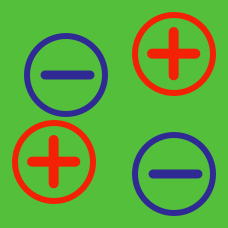Electricity and Magnetism

# Problem solving - charges and fields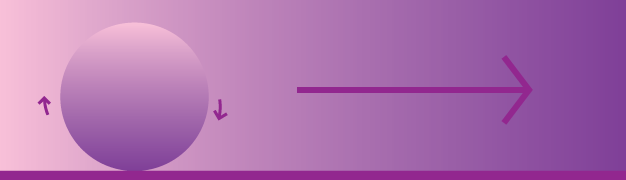A homogeneous ball with mass $$m=1 ~\mbox{g}$$ and total charge $$q=10^{-3}~\mbox{C}$$ (uniformly distributed) is placed on a horizontal $$xy$$-plane. The ball starts rolling without slipping under the influence of a uniform electrostatic field $$\vec{E}=(E,0,0)$$ with $$E=1~ \mbox{V/m}.$$ Find the acceleration in meters per second squared of the center of mass of the ball.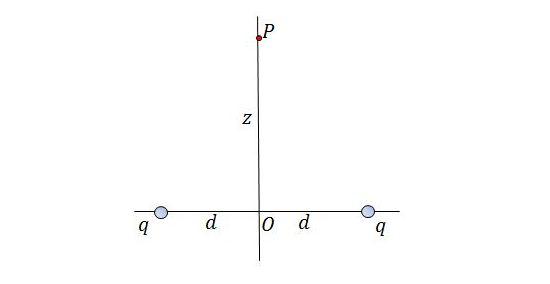If a point charge $$Q = -3 \text{ C }$$ with mass $$m = 1 \text{ kg}$$ is put at point $$P$$ which is at a distance of $$z = 9 \text{ m}$$ above the midpoint between two equal charges $$q = 25 \times 10^{-12} \text{ C },$$ at a distance of $$2d =24 \text{ m}$$ apart, the point charge will be move to point $$O.$$ If the speed of the point charge at point $$O$$ can be expressed as $$v = \sqrt{\frac{a}{b}} \text{ m/s},$$ where $$a$$ and $$b$$ are coprime positive integers, what is the value of $$a+b?$$

Let $$\pi \approx 3,$$ and the permitivity of free space $$\epsilon_0 \approx 9\times 10^{-12} \frac{\text{C}^2}{\text{N*m}^2}.$$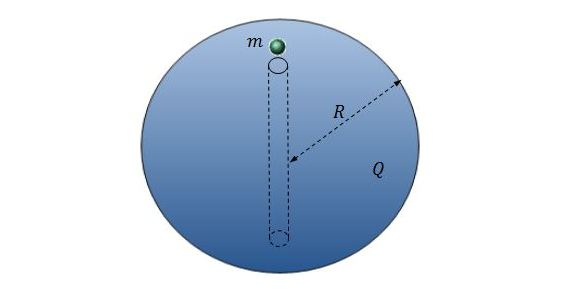There is a point charge with mass $$m = 1 \text{ kg}$$ and charge $$q = -9\times 10^{-6} \text{ C }$$ on a uniformly charged sphere with charge $$Q = 9\times 10^{-6} \text{ C }$$ and radius $$R = 1 \text{ m}$$. As shown in the above diagram, there is a hole on the sphere, so the point charge can move into the hole. If the period of the point charge can be expressed as $$T = 2\pi \sqrt{\frac{a}{b}} \text{ s},$$ where $$a$$ and $$b$$ are coprime positive integers, what is the value of $$a+b?$$

Let $$\pi \approx 3,$$ and the permitivity of free space $$\epsilon_0 \approx 9\times 10^{-12} \frac{\text{C}^2}{\text{Nm}^2}.$$

A point particle with mass $$m = 1 \text{ kg}$$ and charge $$q = -7\times 10^{-6} \text{ C }$$ is rotating around another point particle with charge $$Q = 7\times 10^{-6} \text{ C }$$,which is fixed at the center, with radius $$r = 2 \text{ m}$$. If the speed of the circular motion can be expressed as $$v = \sqrt{\frac{a}{b}} \text{ m/s},$$ where $$a$$ and $$b$$ are coprime positive integers, what is the value of $$a+b?$$

Let $$\pi \approx 3,$$ and the permitivity of free space $$\epsilon_0 \approx 9\times 10^{-12} \frac{\text{C}^2}{\text{N*m}^2}.$$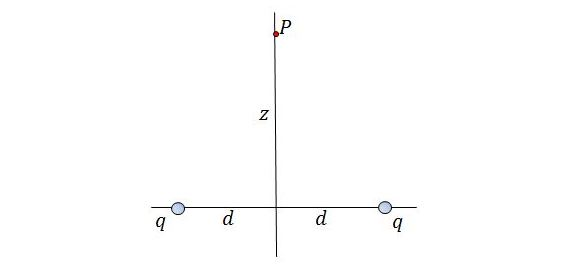The potential at point $$P$$ which is at a distance of $$z = 6 \text{ m}$$ above the midpoint between two equal charges $$q = 500 \times 10^{-12} \text{ C }$$, at a distance of $$2d =16 \text{ m}$$ apart, can be expressed as $$U = \frac{a}{b} \text{ J/C},$$ where $$a$$ and $$b$$ are coprime positive integers. What is the value of $$a+b?$$

Let $$\pi \approx 3,$$ and the permitivity of free space $$\epsilon_0 \approx 9\times 10^{-12} \frac{\text{C}^2}{\text{N*m}^2}.$$

×